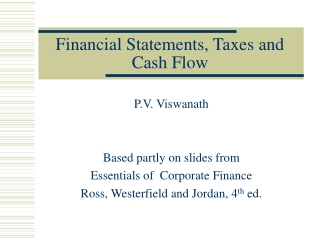DownloadDownload PresentationFinancial Statements, Taxes and Cash Flow

# Financial Statements, Taxes and Cash Flow

Download Presentation## Financial Statements, Taxes and Cash Flow

- - - - - - - - - - - - - - - - - - - - - - - - - - - E N D - - - - - - - - - - - - - - - - - - - - - - - - - - -
##### Presentation Transcript

1. Financial Statements, Taxes and Cash Flow P.V. Viswanath Based partly on slides from Essentials of Corporate Finance Ross, Westerfield and Jordan, 4th ed.

2. Key Concepts and Skills • Know the difference between book value and market value • Know the difference between accounting income and cash flow • Know the difference between average and marginal tax rates • Know how to determine a firm’s cash flow from its financial statements P.V. Viswanath

3. Chapter Outline • The Balance Sheet • The Income Statement • Taxes • Cash Flow P.V. Viswanath

4. The Balance Sheet • The balance sheet is a snapshot of the firm’s assets and liabilities at a given point in time • Assets are listed in order of liquidity • Ease of conversion to cash • Without significant loss of value • Balance Sheet Identity • Assets = Liabilities + Stockholders’ Equity P.V. Viswanath

5. Figure 2.1 P.V. Viswanath

6. US Corporation Balance Sheet – Table 2.1 P.V. Viswanath

7. Market vs. Book Value • The balance sheet provides the book value of the assets, liabilities and equity. • Market value is the price at which the assets, liabilities or equity can actually be bought or sold. • Market value and book value are often very different. Why? • Which is more important to the decision-making process? P.V. Viswanath

8. Klingon Corporation P.V. Viswanath

9. Income Statement • The income statement is more like a video of the firm’s operations for a specified period of time. • You generally report revenues first and then deduct any expenses for the period • Matching principle – GAAP say to show revenue when it accrues and match the expenses required to generate the revenue P.V. Viswanath

10. Table 2.2 P.V. Viswanath

11. Taxes • The one thing we can rely on with taxes is that they are always changing • Marginal vs. average tax rates • Marginal – the percentage paid on the next dollar earned • Average – the tax bill / taxable income • Other taxes P.V. Viswanath

12. Example: Marginal Vs. Average Rates • Suppose your firm earns \$4 million in taxable income. • What is the firm’s tax liability? • What is the average tax rate? • What is the marginal tax rate? • If you are considering a project that will increase the firm’s taxable income by \$1 million, what tax rate should you use in your analysis? P.V. Viswanath

13. The Concept of Cash Flow • Cash flow is one of the most important pieces of information that a financial manager can derive from financial statements • The statement of cash flows does not provide us with the same information that we are looking at here • We will look at how cash is generated from utilizing assets and how it is paid to those that finance the purchase of the assets P.V. Viswanath

14. Cash Flow From Assets • Cash Flow From Assets (CFFA) = Cash Flow to Creditors + Cash Flow to Stockholders • Cash Flow From Assets = Operating Cash Flow – Net Capital Spending – Changes in NWC P.V. Viswanath

15. Example: US Corporation • Operating Cash Flow (I/S) = EBIT + depreciation – taxes = \$547 • Net Capital Spending ( B/S and I/S) = ending net fixed assets – beginning net fixed assets + depreciation = \$130 • Changes in Net Working Capital (B/S) = ending NWC – beginning NWC = \$330 • Cash Flow From Assets (CFFA) = 547 – 130 – 330 = \$87 • CF to Creditors (B/S and I/S) = interest paid – net new borrowing = \$24 • CF to Stockholders (B/S and I/S) = dividends paid – net new equity raised = \$63 • CFFA = 24 + 63 = \$87 P.V. Viswanath

16. Table 2.5 P.V. Viswanath

17. Example: Balance Sheet and Income Statement Information • Current Accounts • 2001: Current Assets = 4500; Current Liabilities = 1300 • 2002: CA = 2000; CL = 1700 • Fixed Assets and Depreciation • 2001: Net Fixed Assets = 3000; 2002: NFA = 4000 • Depreciation expense = 300 • LT Liabilities and Equity • 2001: Long Term Debt = 2200; Common Equity = 500; Retained Earnings = 500 • 2002: LTD = 2800; Common Equity = 750; RE = 750 • Income Statement Information • EBIT = 2700; Interest Expense = 200; Taxes = 1000; Dividends = 1250 P.V. Viswanath

18. Example: Cash Flows • Operating Cash Flow = 2700 + 300 – 1000 = 2000 • Net Capital Spending = 4000 – 3000 + 300 = 1300 • Changes in Net WC = (2000 – 1700) – (1500 – 1300) = 100 • CF From Assets = 2000 – 1300 – 100 = 600 • CF to Creditors = 200 – (2800 – 2200) = -400 • CF to Stockholders = 1250 – (750 – 500) = 1000 • CFFA = -400 + 1000 = 600 • The CF identity holds. P.V. Viswanath

19. Quick Quiz • What is the difference between book value and market value? Which should we use for decision making purposes? • What is the difference between accounting income and cash flow? Which do we need to use when making decisions? • What is the difference between average and marginal tax rates? Which should we use when making financial decisions? • How do we determine a firm’s cash flows? What are the equations and where do we find the information? P.V. Viswanath